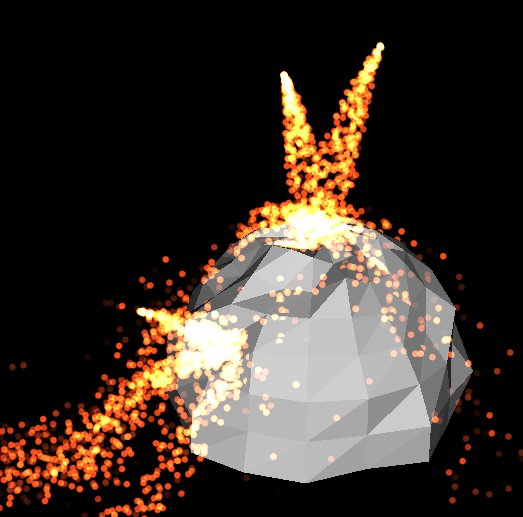# Task gpu008: Particle system in CUDA

Your task is to implement simple particle system in CUDA/OpenCL/GLSLComputeShader . Particles should move in homogenous gravitational field, collide with simple obstacles (represented as triangle meshes) but need not interact with each other. Simple realtime rendering in OpenGL, best using GL_PONTS for particles.There are two options: you can start with the sample14 project from the ogl repository (CUDA in C++) or with the 090opencl project from the grcis repository (OpenCL in C#).

### Details

Each working thread could have its particles in local memory (registers), obstacle (=scene) definition should be in the shared memory. Particle generator[s] implementation may remain in CPU, but it is not difficult to implement generator in CUDA as well..
Use CUDA-OpenGL interop to directly write particle coordinates from CUDA kernel to pre-allocated VBO buffer for rendering.
Particle-triangle intersection: create small line segment using current particle's position and its velocity vector. Intersect the line with a triangle. Bounce the particle in case of collision. You should be able to handle up to 500 triangles w/o problems (see max-shared-memory size).

Example of triangle-segment intersection in CUDA C:
```#define EPSILON     0.0001f
#define MINIEPSILON 0.00001f

struct STriangleCUDA
{
float3 a,b,c;
float3 edge1, edge2, normal;
};

__device__ bool IntersectTriangle ( STriangleCUDA *tri, float3 orig, float3 dir, float *t )
{
const float3 pvec = make_float3( dir.y*tri->edge2.z - dir.z*tri->edge2.y, // dir ^ tri.edge2;
dir.z*tri->edge2.x - dir.x*tri->edge2.z,
dir.x*tri->edge2.y - dir.y*tri->edge2.x );
const float det = tri->edge1.x*pvec.x + tri->edge1.y*pvec.y + tri->edge1.z*pvec.z; // tri.edge1 * pvec;
if( fabs(det) < EPSILON )
return false;

const float inv_det = 1.0f / det;

const float3 tvec = make_float3( orig.x - tri->a.x,
orig.y - tri->a.y,
orig.z - tri->a.z ); // orig - tri.a;
float lambda = tvec.x*pvec.x + tvec.y*pvec.y + tvec.z*pvec.z; // tvec * pvec;
lambda *= inv_det;

if ( lambda < -MINIEPSILON ||
lambda > 1.0f + MINIEPSILON )
return false;

const float3 qvec = make_float3( tvec.y*tri->edge1.z - tvec.z*tri->edge1.y, // tvec^tri.edge1;
tvec.z*tri->edge1.x - tvec.x*tri->edge1.z,
tvec.x*tri->edge1.y - tvec.y*tri->edge1.x );
float mue = dir.x*qvec.x + dir.y*qvec.y + dir.z*qvec.z; // dir * qvec;
mue *= inv_det;
if ( mue < MINIEPSILON ||
mue+lambda > 1.0f + MINIEPSILON )
return false;

float f = tri->edge2.x*qvec.x + tri->edge2.y*qvec.y + tri->edge2.z*qvec.z; // tri.edge2 * qvec;
f *= inv_det;
if ( *t <= f ||
f < EPSILON )
return false;

*t = f;
return true;
}
```

### Interactivity, appearance

Use previously implemented interactivity (trackball). Render both particles and the scene (obstacles), you can implement some fancy aging mechanism for particles - changing color, point-size, etc.

Send complete Visual Studio project including shaders and CUDA/OpenCL source. Write a brief document about your ideas and operating manual.

Hand in before: 3. 7. 2016

### Points

8 points: basic particle system implementation on the CPU (including collision with obstacles and gravitational field),
18 points: the same in CUDA/OpenCL/compute-shaders,
5 points: efficiency study (discuss grid/block size, time measurements..),
8 points: using shared memory for obstacles and/or generators,
4 points: all the data live in the GPU memory (OpenGL interop is used for rendering),
2 points: for each additional collision geometry type (sphere, rectangle, .. max 6 points),
up to 8 points: bonus for nice scene, more forces, fancy particle generators, ..

Attention: you have to implement at least CUDA/OpenCL/shaders option!

### Project

Recommended starting point: Visual Studio project 090opencl from the grcis repository (OpenCL) or the sample14 from the ogl repository (CUDA).

Copyright (C) 2011-2016 J. Pelikán, last change: 2019-05-09 17:52:59 +0200 (Thu, 09 May 2019)